• Markdown是一种可以使用普通文本编辑...我们在写博客的时候，会有想要写公式的情况，这时候我们怎么把公式漂亮的写在博客里面呢？下面介绍三种还算方便的方法： Online LaTex Markdown中的公式语法是遵循LaTex的...
Markdown是一种可以使用普通文本编辑器编写的标记语言，通过简单的标记语法，它可以使普通文本内容具有一定的格式。  在很多主流的博客网站，github等地方都会用到Markdown的语法，比如CSDN。我们在写博客的时候，会有想要写公式的情况，这时候我们怎么把公式漂亮的写在博客里面呢？下面介绍三种还算方便的方法：
Online LaTex
Markdown中的公式语法是遵循LaTex的，所以我们可以用Online Latex这款在线的转化工具把我们想要的数学公式转化成LaTex的语法：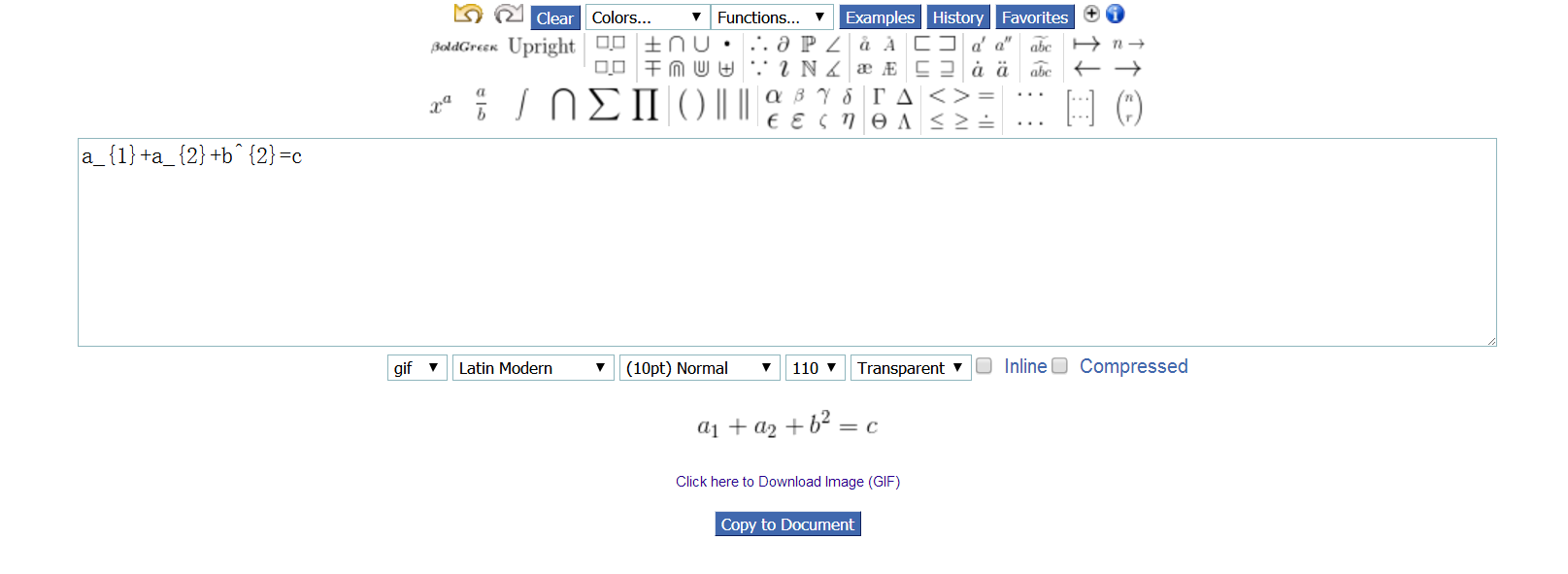比如我们想要一个这样的公式，只需要根据数学符号在编辑框上方选择，然后对应的LaTex语法就会出现在编辑框中，而编辑框的下方，就是我们想要的公式的数学表达。  然后，把编辑框中的公式粘过来，放到之间，就有了下面的效果：

a1+a2+b2=c

a

1

+

a

2

+

b

2

=

c

a_{1}+a_{2}+b^{2}=c

MathType
MathType应该是大多数都在用的公式编辑工具了，MathType软件中同样提供了公式到LaTex代码的转换，在复制选项中：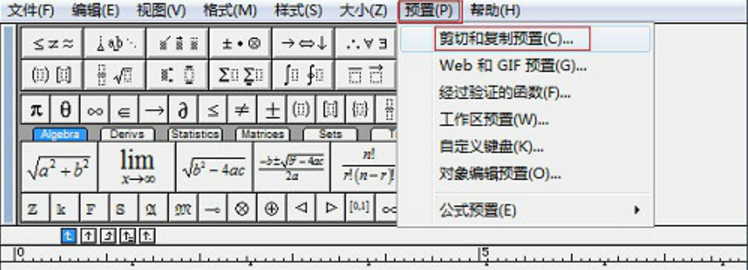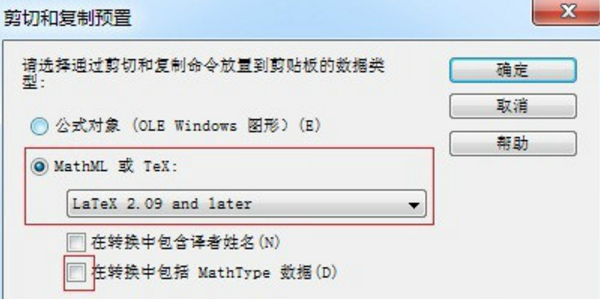LaTex语法
前面两种方法，都是使用了其他工具帮助我们转化成LaTex的代码，显然我们也可以直接在Markdown直接写的，下面我们简单介绍下他的语法。
公式位置：  在行内的公式写在内，行间的公式写在内，基本符号和运算符可以直接使用键盘敲出来，如a+b=c等等：

a+b=c

a

+

b

=

c

a+b=c

上标下标：  a_{}：

a2

a

2

$a_{2}$  a^{}：

a3

a

3

$a^{3}$
括号：  LaTex的括号都在遵循一种结构：\left后面时左括号形式，\right后面时右括号形式，左括号后面的内容就是要写入到括号里面的内容，如：
\left ( \right )：

()

(

)

$\left ( \right )$  \left [ \right ]：

[]

[

]

$\left [ \right ]$  \left { \right }：

{}

{

}

$\left \{ \right \}$  \left | \right |：

||

|

|

$\left | \right |$  \left ( \left ( \right ) \right )：

(())

(

(

)

)

$\left ( \left ( \right ) \right )$
希腊字母：  公式中一般会有大量希腊字母的，这些字母没办法直接用键盘敲出来，如：
\alpha：

α

α

$\alpha$  \beta：

β

β

$\beta$  \gamma：

γ

γ

$\gamma$  \Phi ：

Φ

Φ

$\Phi$  \Omega：

Ω

Ω

$\Omega$  \Delta：

Δ

Δ

$\Delta$  \delta：

δ

δ

$\delta$
其他运算符：  除了常规的加、减、乘、除和等于符号，我们一般还有用到一些其他的运算符，如：
\sum ：

∑

∑

$\sum$  \int ：

∫

∫

$\int$  \oint ：

∮

∮

$\oint$  \prod：

∏

∏

$\prod$
用上面这些东西，我们可以完成一些常见的公式编辑工作，更多LaTex语法请参考 [这儿]。
展开全文文本编辑 markdown
• 实现在matlab标题中，或者标注中写公式，可以采取下面方式： legend('p_{m}^{min}=0.4','p_{m}^{min}=0.5','p_{m}^{min}=0.6'); {}里是下标和上标 实现的效果： ...
实现在matlab标题中，或者标注中写公式，可以采取下面方式：
legend('p_{m}^{min}=0.4','p_{m}^{min}=0.5','p_{m}^{min}=0.6');
{}里是下标和上标
实现的效果：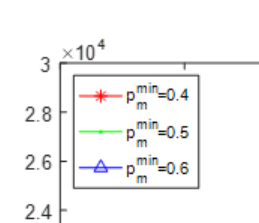展开全文matlab
前言
准备工作
过程
方法一：结合Notes
利用原生的Notes在局域网内能够同步Mac和iPad的功能，我们在iPad上直接进行撰写。在Notes上直接进行撰写，PC端会同步iPad上的内容，但是延迟比较高，大概会延迟十多秒。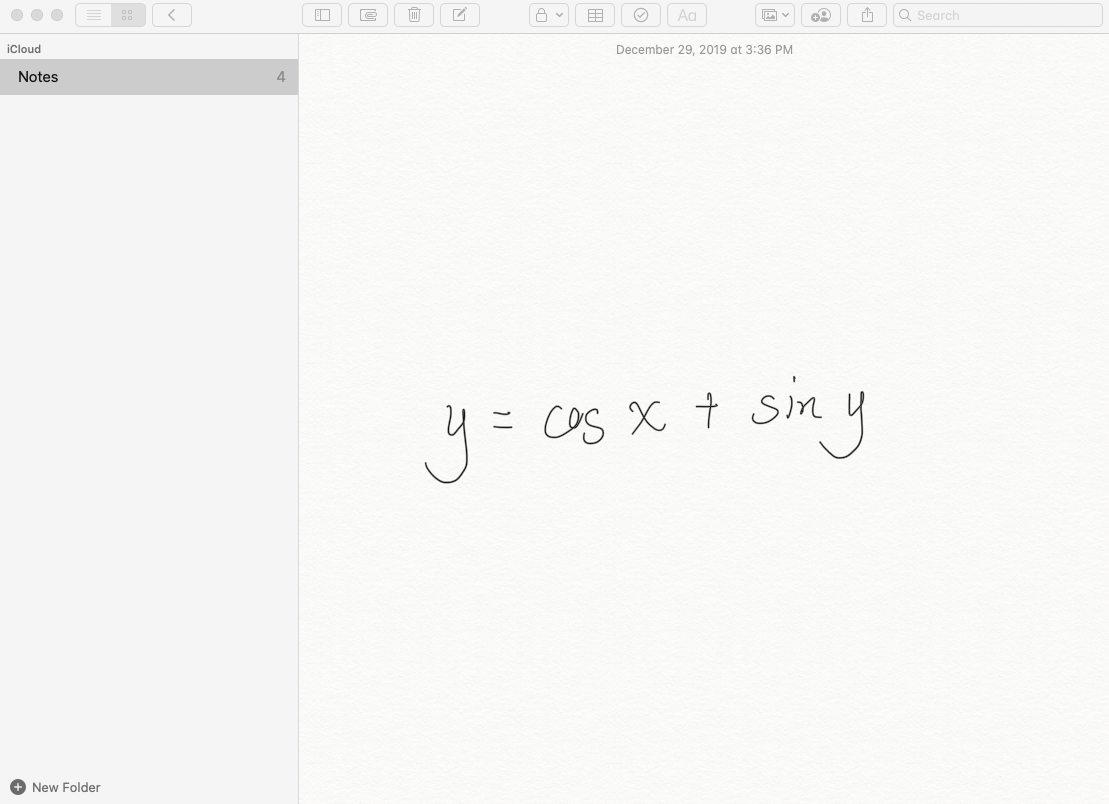然后使用Mathpix Snip进行截图就可以对手写的公式进行识别了。识别完成后可以直接生成LaTeX的公式。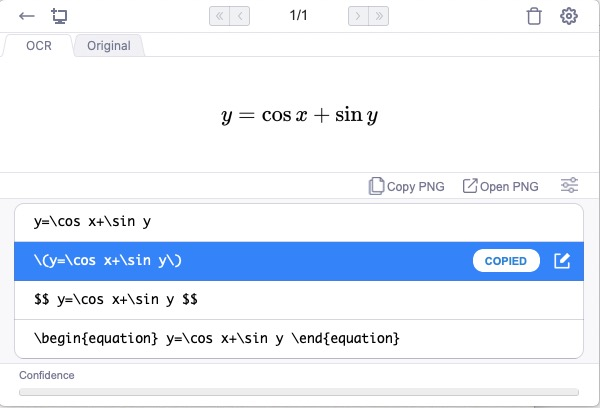方法二：结合OneNote+Sidecar
因为上面的方法延迟比较高，在同一局域网内就可以利用MacOS 10.15的最新功能Sidecar，完成高效率的同步。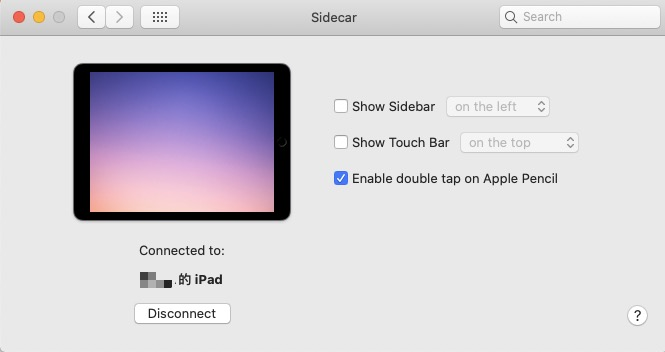这里选用OneNote的原因在于，OneNote是Mac端手写功能较好的且免费的软件。我们将iPad作为Mac的扩展屏，然后Pencil就可以作为鼠标在iPad屏幕上进行书写。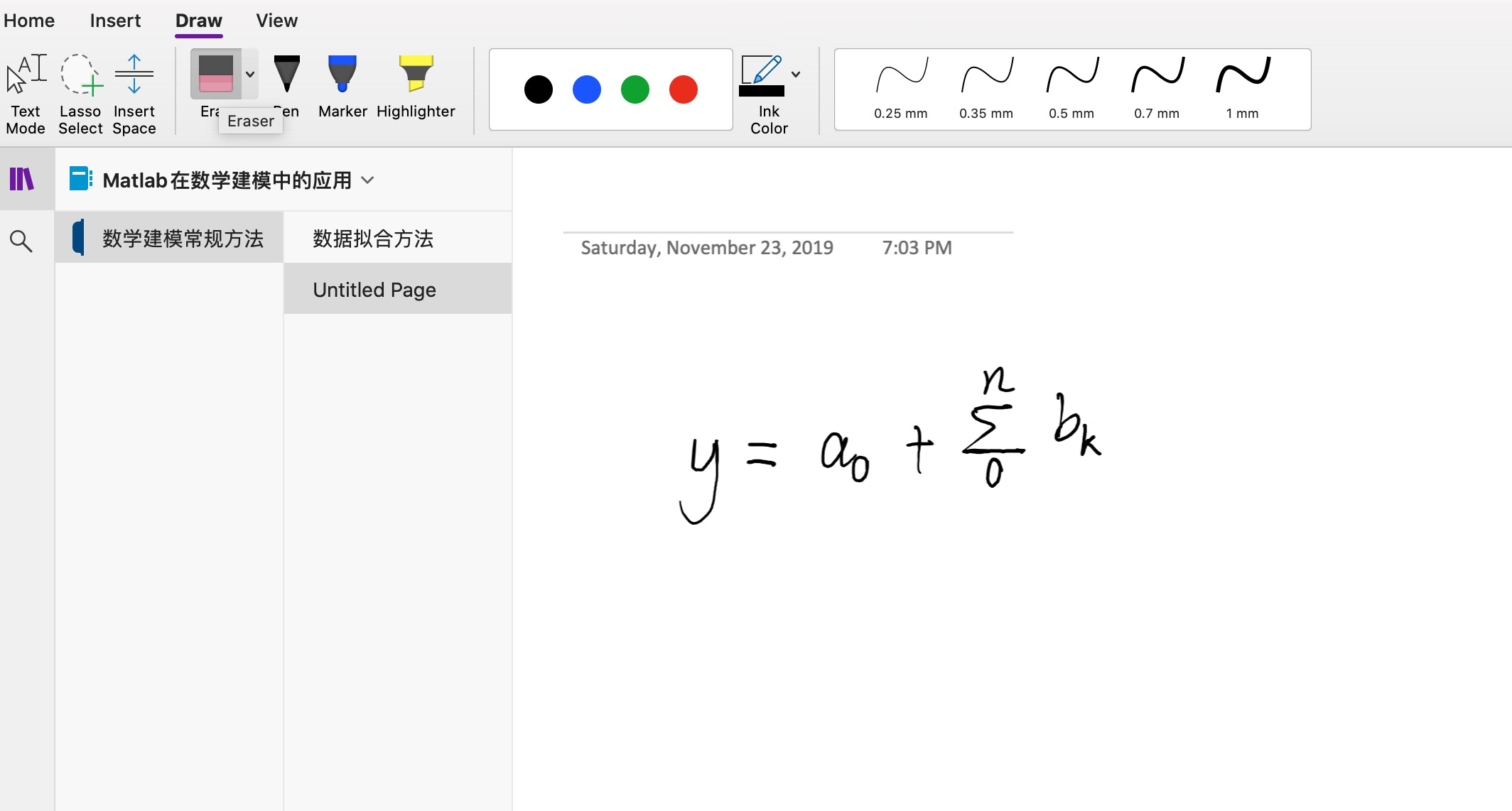完成撰写之后就可以直接进行识别了。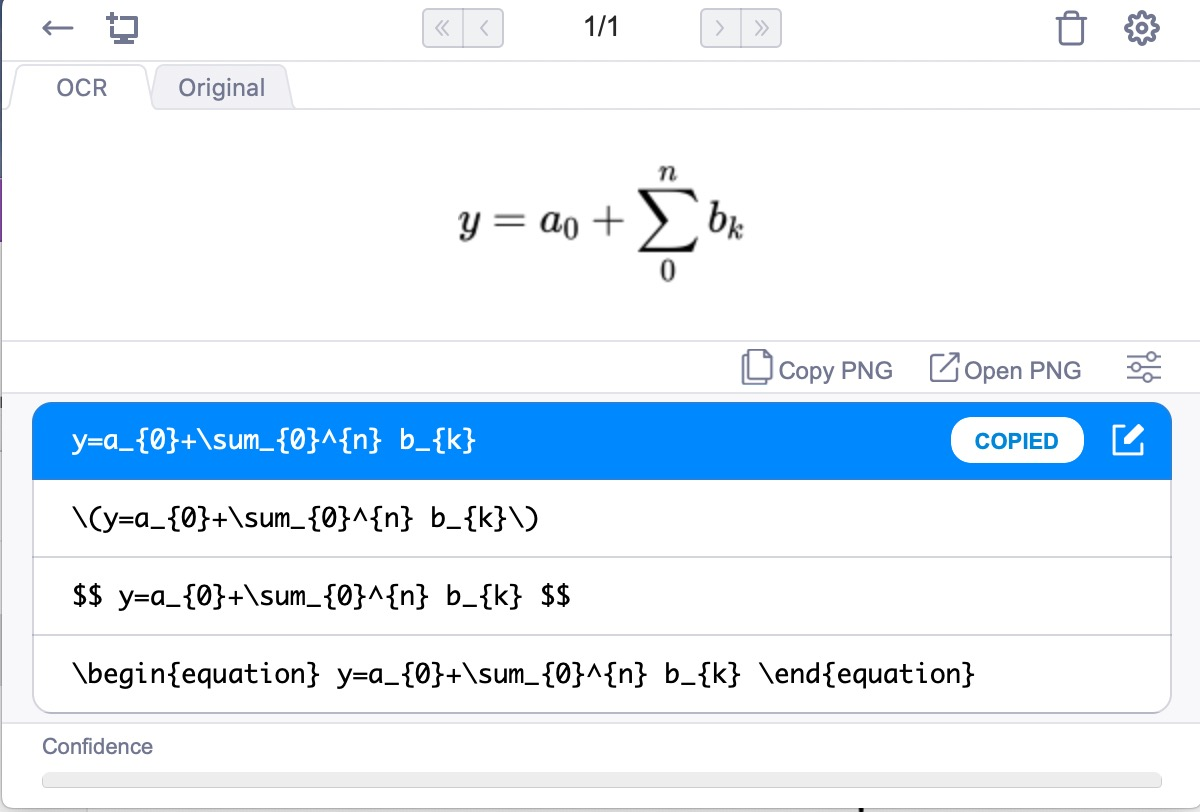展开全文• ## 使用Java写公式计算器

万次阅读 热门讨论 2018-12-15 16:20:07
我的一位好友需要软件里面内置一个小插件，可以根据用户输入的简单公式引导用户进行输入和计算，所以简单地了一个原理实现。主要用到Java字符串处理和数据结构的栈思想，难度不大但要非常细心。 原理如下： 1、...
我的一位好友需要软件里面内置一个小插件，可以根据用户输入的简单公式引导用户进行输入和计算，所以简单地写了一个原理实现。主要用到Java字符串处理和数据结构的栈思想，难度不大但要非常细心。
原理如下：
1、对公式以等号为分隔符进行左右分割，然后取得公式右边字符串
2、对公式右边字符串进行运算符（+、-、*、/、（、））和常数的去除，然后分割出变量名
3、请求用户输入各变量的值，用用户输入值取代字符串的对应变量，使得公式变成纯数字和纯运算符构成的字符串。
4、最复杂的一环：寻找第一个右括号（想到于找到栈顶），然后倒过来找左括号（如公式格式正确则必然同时是最后一个左括号），左括号和右括号作为一个整体提取作为子字符串。子字符串里面先找乘法和除法的子子字符串进行计算，再找加法和除法的子子字符串进行计算（按运算符查找，并找到符号两边的数值），然后运算完之后替换回去作为新的子字符串，直到子字符串没有运算符则结束。
5、子字符串为括号内部运算的最终结果，用该结果替换整个子字符串，调到第1步开始重复。直到公式没有一个运算符停止

实现代码：
package com.test.calc;

import java.util.HashMap;
import java.util.List;
import java.util.Map;
import java.util.Scanner;
import java.util.Stack;
import java.util.function.Consumer;

/**公式计算器**/
public class EquationCalculator {

/** 变量集合 **/
private static List<String> varList = new LinkedList<>();
/** 变量和值的key-value **/
private static Map<String, Float> varListWithValue = new HashMap<>();

public static void main(String[] args) {
Scanner scanner = new Scanner(System.in);
System.out.println("请输入你需要计算的公式:");
String formula = scanner.nextLine().replaceAll(" ", "");
//String formula = "f=k * (((m1 + m2) * (40 * x + 60 * y)) + 100) * m2 / (G1 + G2)".replaceAll(" ", ""); fortest
String equalationLeft = formula.split("=");
String equalationRight = formula.split("=");
/** 获取变量表: **/
getVarList(equalationRight);
/** 给变量赋值 **/
assignmentVarList(varList);
/** 代回公式进行替换 **/
String newFormula = replaceEquation(formula);
/** 进行计算 **/
System.out.println();
float result = calc(newFormula);
System.out.println("最终结果:" + equalationLeft + "=" + result);
}

/** 进行计算 **/
private static float calc(String newFormula) {
boolean stillHaveCalcSymbol = false;
do{
//System.out.println("before:" + newFormula);
/** 寻找最后一个左括号里面到第一个右括号里面1的内容 **/
char formulaArray[] = newFormula.toCharArray();
for (int i = 0; i < formulaArray.length; i++) {
if (formulaArray[i] == '+' || formulaArray[i] == '-'
|| formulaArray[i] == '*' || formulaArray[i] == '/'
|| formulaArray[i] == '(' || formulaArray[i] == ')') {
stillHaveCalcSymbol = true;
} else {
stillHaveCalcSymbol = false;
}
}
if (stillHaveCalcSymbol) {
String resultFormula = "";
//找最内层的括号里面的内容出来（含括号）
for (int i = 0; i < formulaArray.length; i++) {
if (formulaArray[i] == ')') {
int begin = 0;
for (int j = i; j >= 0; j--) {
if (formulaArray[j] == '(') {
begin = j;
break;
}
}
String calcString = newFormula.substring(begin, i + 1);
resultFormula = newFormula.replace(calcString, calcProc(calcString) + "");
//System.out.println(calcString);
break;
}
}
newFormula = resultFormula;
}
} while(stillHaveCalcSymbol);
//最后得到普通的顺序无括号公式：
System.out.println(newFormula);
//最后一次计算:
float result = calcProc("(" + newFormula.split("=") + ")");
return result;
}

/**详细计算过程**/
private static float calcProc(String calcString) {
//		if(calcString.contains("=")){
//			calcString = calcString.split("=");
//		}
//calcString = calcString.replace("(", "");
//calcString = calcString.replace(")", "");

String calcSymbol[] = {"\\*", "\\/", "\\+", "\\-"};
char calcSymbolChar[] = {'*', '/', '+', '-'};
boolean haveSymbol = true;
float result = 0f;
while(haveSymbol){
System.out.println("calcStr:" + calcString);
char calcCharArr[] = calcString.toCharArray();
result = 0f;
for (int i = 0; i < calcSymbol.length; i++) {
for(int j = 0; j < calcCharArr.length; j++){
if(calcCharArr[j] == calcSymbolChar[i]){
//System.out.println("找到了" + calcSymbolChar[i]);
//以符号为中心，以左右两边的其他符号为边界找到两边的数
float num1 = 0f;
float num2 = 0f;
int bottom = 0;
for(int k = j - 1; k >= 0 && (calcCharArr[k] >= '0' && calcCharArr[k] <= '9' || calcCharArr[k] == '.') ; k--){
//System.out.println(calcCharArr[k] + "");
bottom = k;
}
//System.out.println("[j, bottom]:" + String.format("[%d, %d]", j, bottom));
num1 = Float.valueOf(calcString.substring(bottom, j));
System.out.println("num1:" + num1);
int top = 0;
for(int k = j + 1; k < calcString.length() && (calcCharArr[k] >= '0' && calcCharArr[k] <= '9' || calcCharArr[k] == '.'); k++){
top = k;
}
num2 = Float.valueOf(calcString.substring(j + 1, top + 1));
System.out.println("num2:" + num2);
switch(calcSymbolChar[i]){
case '*':
result = num1 * num2;
break;
case '/':
result = num1 / num2;
break;
case '+':
result = num1 + num2;
break;
case '-':
result = num1 - num2;
break;
}
//System.out.println("bottom to top:" + calcString.substring(bottom + 1, top + 1));
calcString = calcString.replace(calcString.substring(bottom, top + 1), String.format("%.5f", result));
//System.out.println("end_calcStr:" + calcString);
break;
}
}
}
haveSymbol = false;
if(calcString.contains("*") || calcString.contains("/") || calcString.contains("+") || calcString.contains("-")){
haveSymbol = true;
//System.out.println("找到");
} else {
//System.out.println("找不到");
}
}
//System.out.println("result:" + result);
return result;
}

/** 代回公式进行替换 **/
private static String replaceEquation(String formula) {
String newFormula = new String(formula);
for (String key : varList) {
newFormula = newFormula.replaceAll(key, varListWithValue.get(key)
+ "");
}
System.out.println(newFormula);
return newFormula;
}

/**
* 给变量赋值
*
* @param varList
*            变量列表
**/
private static void assignmentVarList(List<String> varList) {
System.out.println("请输入各变量的对应值");
Scanner scanner = new Scanner(System.in);
for (String key : varList) {
System.out.print(key + "'s value is:");
varListWithValue.put(key, scanner.nextFloat());
}
/*for (String key : varList) {
System.out.println("key:" + key + ", value:"
+ varListWithValue.get(key));
}*/
}

/**
* 获取变量表
*
* @param equalationRight
*            输入等式的右边
**/
private static void getVarList(String equalationRight) {
System.out.println("等式右边:" + equalationRight);
char[] formulaCharArr;
formulaCharArr = equalationRight.toCharArray();
//清理所有运算符
for (int i = 0; i < formulaCharArr.length; i++) {
if (formulaCharArr[i] == '+' || formulaCharArr[i] == '-'
|| formulaCharArr[i] == '*' || formulaCharArr[i] == '/'
|| formulaCharArr[i] == '(' || formulaCharArr[i] == ')') {
formulaCharArr[i] = ' ';
}
}
/*String temp = "";
for (int i = 0; i < formulaCharArr.length; i++) {
if (formulaCharArr[i] == ' ') {
String content = temp.trim();
if (content.length() > 0) {
boolean okGo = true;
if(content.charAt(0) >= '0' && content.charAt(0) <= '9'){
okGo = false;
}
if (okGo) {
}
}
temp = "";
} else {
temp += formulaCharArr[i];
}
}*/
String pa[] = new String(formulaCharArr).split(" ");
for(String temp : pa){
if(temp != null && temp != "" && !temp.isEmpty()){
boolean okGo = true;
if(temp.charAt(0) >= '0' && temp.charAt(0) <= '9'){
okGo = false;
}
if (okGo) {
}
}
}
System.out.println("变量列表:");
for (int h = 0; h < varList.size(); h++) {
String var = varList.get(h);
System.out.println(var);
}
}

}


测试1：
请输入你需要计算的公式: j=b*y+a 等式右边:b*y+a 变量列表: b y a 请输入各变量的对应值 b's value is:55 y's value is:66 a's value is:77 j=55.0*66.0+77.0
j=55.0*66.0+77.0 calcStr:(55.0*66.0+77.0) num1:55.0 num2:66.0 calcStr:(3630.00000+77.0) num1:3630.0 num2:77.0 最终结果:j=3707.0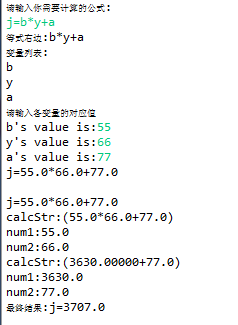测试2：
请输入你需要计算的公式: f=k * (((m1 + m2) * (40 * x + 60 * y)) + 100) * m2 / (G1 + G2) + (G3 * G4) 等式右边:k*(((m1+m2)*(40*x+60*y))+100)*m2/(G1+G2)+(G3*G4) 变量列表: k m1 m2 x y m2 G1 G2 G3 G4 请输入各变量的对应值 k's value is:1.1 m1's value is:2.232 m2's value is:3.333 x's value is:4.567 y's value is:31.44 m2's value is:54.22 G1's value is:5454 G2's value is:111 G3's value is:1112 G4's value is:3333 f=1.1*(((2.232+54.22)*(40*4.567+60*31.44))+100)*54.22/(5454.0+111.0)+(1112.0*3333.0)
calcStr:(2.232+54.22) num1:2.232 num2:54.22 calcStr:(40*4.567+60*31.44) num1:40.0 num2:4.567 calcStr:(182.67999+60*31.44) num1:60.0 num2:31.44 calcStr:(182.67999+1886.40002) num1:182.68 num2:1886.4 calcStr:(56.452*2069.08) num1:56.452 num2:2069.08 calcStr:(116803.71+100) num1:116803.71 num2:100.0 calcStr:(5454.0+111.0) num1:5454.0 num2:111.0 calcStr:(1112.0*3333.0) num1:1112.0 num2:3333.0 f=1.1*116903.71*54.22/5565.0+3706296.0 calcStr:(1.1*116903.71*54.22/5565.0+3706296.0) num1:1.1 num2:116903.71 calcStr:(128594.08594*54.22/5565.0+3706296.0) num1:128594.086 num2:54.22 calcStr:(6972371.50000/5565.0+3706296.0) num1:6972371.5 num2:5565.0 calcStr:(1252.89697+3706296.0) num1:1252.897 num2:3706296.0 最终结果:f=3707549.0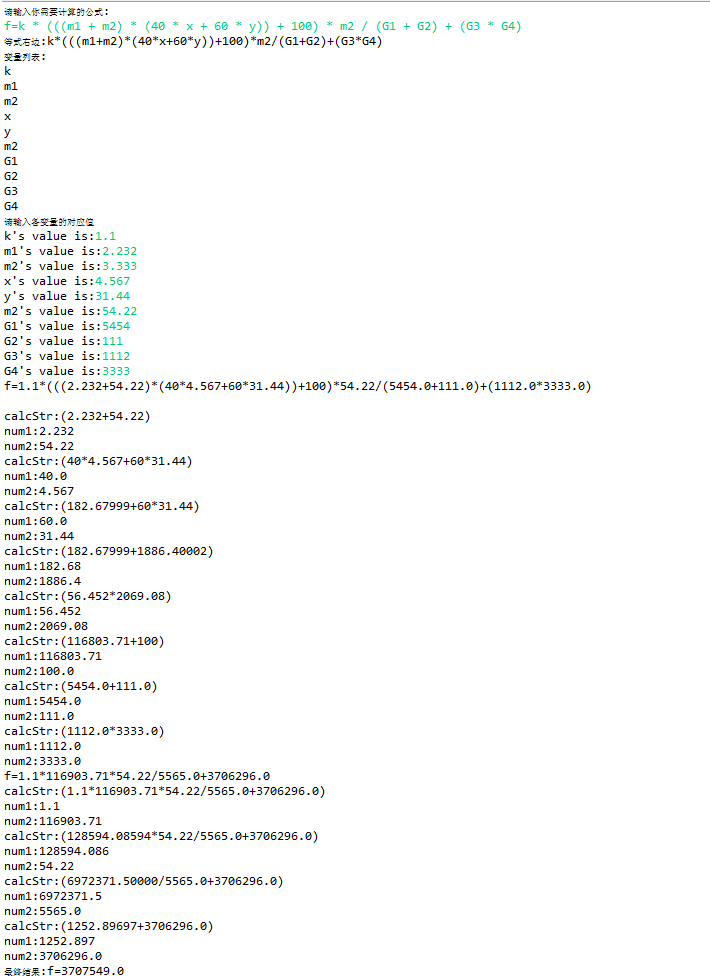展开全文JAVA
• 有道云笔记的markdown编辑器如何通过mathtype来写公式 mathtype设置一下 2. 复制到有道云笔记里稍作修改 在有道云笔记markdown里点插入公式，然后复制我们mathtype写好的公式： 然而提示错误，但是...
• 用LaTeX写公式转换到Word的方法 1. 使用网站 http://engenharialivre.com/latex-para-word/ 输入\frac{\alpha}{\beta} 2.对下方公式右键，转换成MathML语言，弹出窗口，全选复制 3. 打开Word 右键-粘贴...word latex
• Excel写公式工具： 方便公式编写、公式学习，对于不了解公式的人，通过汉字描述自动生成Excel公式。支持Excel多种公式，支持多条件查找公式、多条件求和公式、排名公式、平均值公式、合并单元格公式、提取文字部分...Excel合并
• ## Github写公式

千次阅读 2017-09-13 18:28:13
在文章中添加如下代码 https://cdn.mathjax.org/mathjax/latest/MathJax.js?config=TeX-MML-AM_CHTML"> script> 然后到Latex在线编辑网站 http://www.codecogs.com/latex/eqneditor.php  编写就OK了
• 如何求满足条件的行及列的和（支持合并单元格）---Excel 多条件行、条件列对应的和...点击：Excel自动写公式工具（根据汉字描述写公式,支持合并单元格公式） 点击：汇总满足条件的行及列的和 操作如下图： ...
• 点击：Excel自动写公式工具（根据汉字描述写公式,支持合并单元格公式） 选择下面的其中一个： 查询满足条件的行的数量（个数） 查询满足条件的单元格的数量（个数） 查询满足条件行及列的（满足条件...
• 推荐网站：手写公式转为latex代码 2012年11月26日 ⁄ 论文撰写, 软件使用 ⁄ 暂无评论 ⁄ 被围观 2,106 views+ 看看这个效果，是不是很神奇。 访问如下网址就可以 ...svm latex
• 版权声明：本文为博主原创文章转载请标明地址： ...接下来我就讲一讲怎么这个公式： 1.首先，用公式用\begin{equation}和\end{equation}作为开头和结尾，这样会产生公式并标注（1）序号， ...
• alt和=快捷键调出word自带的公式编辑器 利用引用实现自动编号
• 如何在excel中写公式连接字符串时如何生成单引号和双引号？ 本人在工作中常用到excel来辅助编程。当要做大量重复而有规律的操作时，特别是文本编辑，excel的公式可以起到相当强大的作用，增加工作效率！ 下面介绍...连接字符串 单引号 双引号
• 现在的学习和办公，都离不开电脑，那么也要学会在电脑上编写数学公式，如果纯靠手打的话，那样非常费时间，所以可以借助专业的公式编辑软件。 说到公式编辑软件，推荐大家选择专业的MathType，最新版MathType 7获取...
• $\sum_{x\in X}$ 在某些markdown显示器中呈现角标效果 这时加上\limits标签可以使其呈现下标效果。 $\sum\limits_{x\in X}...或者成单独一行， $$\sum_{x\in X}$$ ∑ x ∈ X \sum_{x\in X} x ∈ X ∑ ​markdown • 由于疫情在家，大学生和研究生都需要自己的论文。使用的工具叫做MathType，它是加拿大的公司开发的，在国内无法购买正版，但是可以使用破解版。 自从Mathtype7的发布，很多的老师和学生都不知道它其实是免费可以... • （1）一般excel都只能在一个单元格，且是输入公式的这个单元格，返回一个值 （2）少数情况，可以在这个一单元格返回多个值 （3）现在考虑的是，输入公式后，可以返回多个值 看起来公式还是遵循了（1）原则 ... • 1.数学太重要了，有些人看论文难啃的原因就是公式看不懂，公式看不懂就是数学不好，后面要慢慢补起来 2.感觉工程能力太重要，不管是在工作中还是面试，算法最终能否实现还是要看你的手上的活．这是我的弱项，慢慢补... • 用cases不能换行，可以采用如下： \begin{equation}\label{equ1} P_r(l_i) = \left\{ \begin{array}{ccc}G_\sigma(1-(\lambda_1-\lambda_2))G_\sigma(\lambda_2-\lambda_3)G_\sigma(\lambda_3), l_i = 1 \\ ... • 用PythonLatex公式——handcalcs工具包 在论文或者做ppt的时候经常需要进行很多的公式推导，通常一些简单的公式我们希望可以通过程序编码直接地实现，并可以导出为pdf供更多的应用。 我个人通常的做法是到一些...python 编程语言 github • 文华财经指标公式期货软件指标公式文华公式指标一位顶尖期货高手公式源码.doc文档 • 最近常常会到数学公式，可是由于不会用LaTeX，出来贼难看。下面分享如何用LaTeX漂亮的数学公式 添加公式的方法 行内公式$行内公式\$ 行间公式 $$行间公式$$ Latex 数学公式语法 角标（上下标） ...latex
• 一位顶尖期货高手的指标公式文华财经指标公式期货软件指标公式文华公式指标.doc文档
• 文华财经指标公式期货软件指标公式文华公式指标一位顶尖期货高手的指标公式.doc文档...